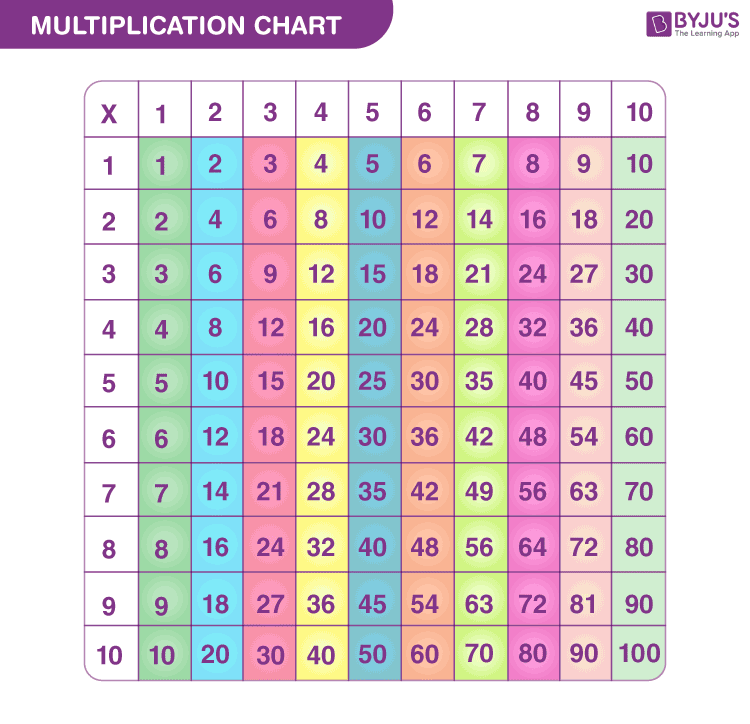Jet Set Go! All about Aeroplanes Jet Set Go! All about Aeroplanes

# Tables 1 to 10

Tables 1 to 10 are the multiplication tables for the numbers from 1 to 10. These tables show the multiples of numbers from 1 to 10. The tables are very beneficial for students, especially for primary and secondary classes.

Learning tables from 1 to 10 will help students to solve multiplication problems in a quick way. Thus, it is very important for students to memorise the tables from 1 to 10.

Get complete tables: Tables 1 to 100

## Maths Tables 1 to 10

Below is the list of multiplication tables from 1 to 10. We have also provided the downloadable PDF for all the tables so that students can download and use it offline.

 Table of 1 Table of 2 Table of 3 Table of 4 Table of 5 1 ×‌ 1 = 1 2 ×‌ 1 = 2 3 × ‌1 = 3 4 × ‌1 = 4 5 × ‌1 = 5 1 ×‌ 2 = 2 2 ×‌ 2 = 4 3 × ‌2 = 6 4 × ‌2 = 8 5 × ‌2 = 10 1 × ‌3 = 3 2 × ‌3 = 6 3 × ‌3 = 9 4 × ‌3 = 12 5 × ‌3 = 15 1 × ‌4 = 4 2 × ‌4 = 8 3 × ‌4 = 12 4 × ‌4 = 16 5 × ‌4 = 20 1 × ‌5 = 5 2 × ‌5 = 10 3 × ‌5 = 15 4 × ‌5 = 20 5 × ‌5 = 25 1 × ‌6 = 6 2 × ‌6 = 12 3 × ‌6 = 18 4 × ‌6 = 24 5 × ‌6 = 30 1 × ‌7 = 7 2 × ‌7 = 14 3 × ‌7 = 21 4 × ‌7 = 28 5 × ‌7 = 35 1 × ‌8 = 8 2 × ‌8 = 16 3 × ‌8 = 24 4 × ‌8 = 32 5 × 8 = 40 1 × ‌9 = 9 2 × ‌9 = 18 3 × ‌9 = 27 4 × ‌9 = 36 5 × 9 = 45 1 × ‌10 = 10 2 × ‌10 = 20 3 × ‌10 = 30 4 × ‌10 = 40 5 × 10 = 50
 Table of 6 Table of 7 Table of 8 Table of 9 Table of 10 6 × 1 = 6 7 × 1 = 7 8 × 1 = 8 9 × 1 = 9 10 × 1 = 10 6 × 2 = 12 7 × 2 = 14 8 × 2 = 16 9 × 2 = 18 10 × 2 = 20 6 × 3 = 18 7 × 3 = 21 8 × 3 = 24 9 × 3 = 27 10 × 3 = 30 6 × 4 = 24 7 × 4 = 28 8 × 4 = 32 9 × 4 = 36 10 × 4 = 40 6 × 5 = 30 7 × 5 = 35 8 × 5 = 40 9 × 5 = 45 10 × 5 = 50 6 × 6 = 36 7 × 6 = 42 8 × 6 = 48 9 × 6 = 54 10 × 6 = 60 6 × 7 = 42 7 × 7 = 49 8 × 7 = 56 9 × 7 = 63 10 × 7 = 70 6 × 8 = 48 7 × 8 = 56 8 × 8 = 64 9 × 8 = 72 10 × 8 = 80 6 × 9 = 54 7 × 9 = 63 8 × 9 = 72 9 × 9 = 81 10 × 9 = 90 6 × 10 = 60 7 × 10 = 70 8 × 10 = 80 9 × 10 = 90 10 × 10 = 100

## Multiplication Chart 1 to 10## Multiplication Tables 2 to 10

Here is the individual list of tables from 2 to 10. Students can directly check the multiplication table of a particular number by clicking on the below links.

## Tables 1 to 10 Solved Examples

Q.1: John has 5 colour boxes. If each box contains 7 colours, then how many total colours are present with John?

Solution: Number of colour boxes = 5

Number of colours in each box = 7

Total number of colours = 5 x 7 = 35

Q.2: A man travels 4 km every day. If he travels for 6 days a week, then how many kilometres does he travel?

Solution: Distance travelled by man in a day = 4Km

Distance travelled by him in 6 days = 4 x 6 = 24 Km

## Practice Questions

1. What is the 9th multiple of 3?
2. What is the value of 7 multiplied by 7?
3. What is the product of 8 and 10?
4. What are 6 times 8?

## Frequently Asked Questions on Tables 1 to 10

### What is the value of 5 times 8?

5 times 8 is equal to 40.
5 x 8 = 40

### What should be multiplied by 4 to get 32?

8 should be multiplied by 4 to get 32.
4 x 8 = 32

### What are 10 times 10?

When 10 is multiplied by 10, then the answer is 100.
10 x 10 = 100

### What is the sum of 3 times 9 and 5 times 2?

3 times 9 is 27 and 5 times 2 is 10. The sum of 27 and 10 is 37.

### What is the value of 9 x 7?

The value of 9 x 7 is 63.